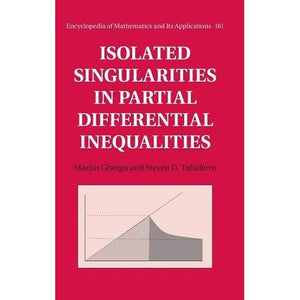># Isolated Singularities in Partial Differential Inequalities (Encyclopedia of Mathematics and its Applications)

• £40.99
• Save £75

Steven D. Taliaferro Marius Ghergu
Cambridge University Press, 2/4/2016
EAN 9781107138384, ISBN10: 1107138388

Hardcover, 362 pages, 24 x 16.5 x 2.7 cm
Language: English

In this monograph, the authors present some powerful methods for dealing with singularities in elliptic and parabolic partial differential inequalities. Here, the authors take the unique approach of investigating differential inequalities rather than equations, the reason being that the simplest way to study an equation is often to study a corresponding inequality; for example, using sub and superharmonic functions to study harmonic functions. Another unusual feature of the present book is that it is based on integral representation formulae and nonlinear potentials, which have not been widely investigated so far. This approach can also be used to tackle higher order differential equations. The book will appeal to graduate students interested in analysis, researchers in pure and applied mathematics, and engineers who work with partial differential equations. Readers will require only a basic knowledge of functional analysis, measure theory and Sobolev spaces.

Preface
1. Representation formulae
2. Isolated singularities of nonlinear elliptic inequalities
3. More on isolated singularities for semilinear elliptic inequalities
4. Elliptic inequalities for the Laplace operator with Hardy potential
5. Singular solutions for nondivergence type elliptic inequalities
6. Isolated singularities of polyharmonic inequalities
7. Nonlinear biharmonic inequalities
8. Initial blow-up for nonlinear parabolic inequalities
9. Semilinear elliptic systems of differential inequalities
10. Isolated singularities for nonlocal elliptic systems
11. Isolated singularities for systems of parabolic inequalities
Appendix A. Estimates for the heat kernel
Appendix B. Heat potential estimates
Appendix C. Nonlinear potential estimates
Bibliography
Index.